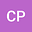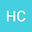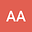BayClump: Bayesian Calibration and Temperature Reconstructions for Clumped Isotope Thermometry
•••• +5
Cristian Román PalaciosUniversity of California -Los Angeles, University of California -Los Angeles

Corresponding Author:cromanpa94@email.arizona.edu

Author ProfileHannah Carroll
University of California -Los Angeles, University of California -Los Angeles
Author ProfileRandy Flores
University of California -Los Angeles, University of California -Los Angeles
Author ProfileQuan Gan
University of Arizona
Author Profile## Abstract

Carbonate clumped isotope thermometry (Δ47) is a temperature proxy that is becoming more widely used in the geosciences. Most calibration studies have used ordinary least squares linear regressions or York models to describe the relationship between Δ47 and temperature. However, Bayesian models have not yet been explored for clumped isotopes. There also has not yet been a comprehensive study assessing the performance of commonly used regression models in the field. Here, we use simulated datasets to compare the performance of seven regression models, three of which are new and fit using a Bayesian framework. All models recover regression parameters within error of true values. Ordinary least squares linear and Bayesian models have the highest precision and accuracy. Congruently, for temperature reconstructions where the fitted model is used to predict temperature from Δ47, Bayesian models generally outperform other regression models in both precision and accuracy. Our analyses suggest that depending on the structure of the examined dataset and relative to classical models, Bayesian regressions could improve the accuracy and precision of (i) calibration parameter estimates and (ii) temperature reconstructions by at least a factor of two. We implement our comparative framework into a new web-based interface, BayClump. This tool should increase reproducibility by enabling access to the different Bayesian and non-Bayesian regression models examined here. We utilize this tool with a published data synthesis to assess regression parameters and show that while both yield similarly accurate results, uncertainty in estimates of the slope and regression are reduced.